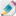# Right angled Trigonometry

##SOHCAHTOA

This part of trigonometry can be seen in the recurring relationships between the sides of right angles triangles. The relationship means that we can use two bits of information about a triangle to work out a third and so on until we know everything we need to know. As such it is a really useful tool both for further mathematics an in a nmber of very practical applications.

The following is a series of slides and videos that will help you understand, learn about and review this sub-topic.

Keep track of your progress and practice the exam questions on thisRight angled trigonometry record sheet

### Slides Gallery

Use these slides to review the material and key points covered in the videos.

####Understanding trig ratios

This video explains the fundamental concept behind trigonometrical relationships.

####Making Statements

Solving problems with trigonometry rests heavily on being able to take a given scenario and make correct statements of equality about it. These statements can then be used to find unknowns.

####Rearranging equations

Often the thing that students find most difficult when solving trig problems is successfully rearranging the equations. This is a really important bit of algebraic manipulation that is useful in lots of places.

####Solving for angles

Here we look at solving right angled trig problems where the unknown is an angle.

####Solving for side lengths

Here we look at solving right angled trig problems where the unknown is an side length.

####Angles of elevation and depression

These are important definitions to be aware of so you dont tae a wrong turn at the beginning of a question.

### Revise

This section of the page can be used for quick review. The flashcards help you go over key points and the quiz lets you practice answering questions on this subtopic.

#### Flash Card

Review this condensed 'key point' flashcard to help you check and keep ideas fresh in your mind.#### Practice Questions

Practice your understanding on these quiz questions. Check your answers when you are done and read the hints where you got stuck. If you find there are still some gaps in your understanding then go back to the videos and slides above. NOTE - Make sure that you have set your GDC to be in degrees. See the  Your Graphical Display Calculator page for help with this.

### Exam Style Questions

The following questions are based on IB exam style questions from past exams. You should print these off (from the document at the top) and try to do these questions under exam conditions. Then you can check your work with the video solution. NOTE - Make sure that you have set your GDC to be in degrees. See the  Your Graphical Display Calculator page for help with this.

.....